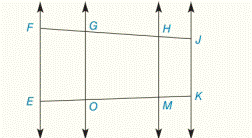Chapter 5.CR, Problem 27CRElementary Geometry For College St...

7th Edition
Alexander + 2 others
ISBN: 9781337614085

Solutions

Chapter
SectionElementary Geometry For College St...

7th Edition
Alexander + 2 others
ISBN: 9781337614085
Textbook Problem

Given: E F ↔ | | G O ↔ | | H M ↔ | | J K ↔ | | , with     transversals     F J ↔     and     E K ↔ ; F G = 2 , G H = 8 , H J = 5 , E M = 6 Find EO, EKTo determine

To find:

EO, EK

Explanation

Given:

EF||GO||HM||JK||FG=2,GH=8,HJ=5,EM=6

Then,

Calculation:

Using, “When two or more parallel lines are cut by a pair of transversal, the transversals are divided proportionally by the parallel lines”.

So,

FGEO=FHEM2EO=2+862EO=106

Cross multiplying, we get

2EO=106(EO)⋅</

Still sussing out bartleby?

Check out a sample textbook solution.

See a sample solution

The Solution to Your Study Problems

Bartleby provides explanations to thousands of textbook problems written by our experts, many with advanced degrees!

Get Started

Find more solutions based on key concepts